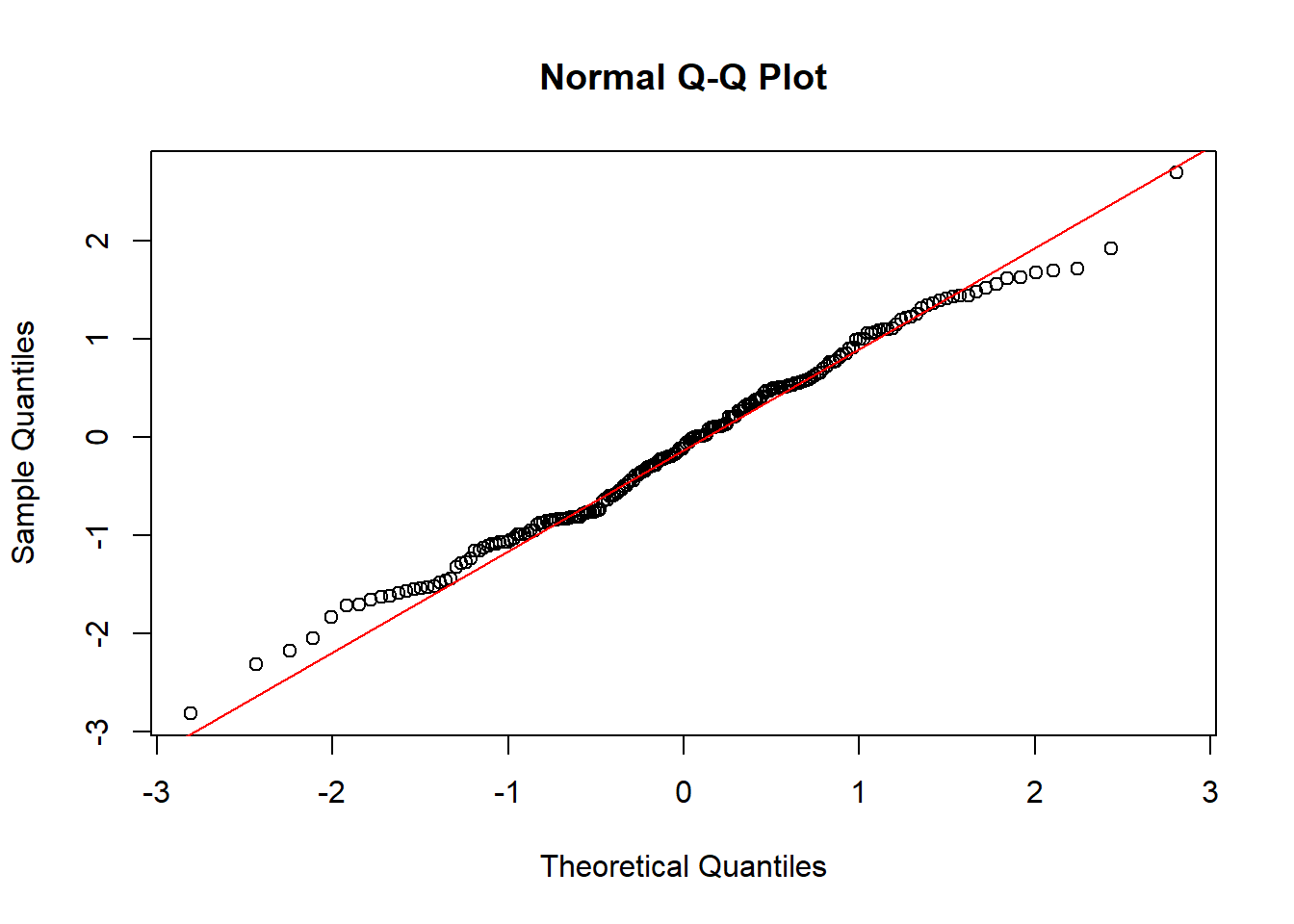# 第 7 章 Goodness of Fit

## 7.1 多项分布

• 多项分布是二项分布的推广。
• 总体被分为几个互不相交的类别。
• 多项分布假设：每次试验有且仅有一个结果发生；每次试验独立；每次试验概率不变。

• 1.计算每一类实际观测到的频次$$f_i$$
• 2.计算每一类理论上的期望频次$$e_i$$
• 3.计算 Chi-square 统计量——$$\chi^2=\sum(f_i-e_i)^2/e_i$$。其中自由度 (df) = k-1， k 是多项总体的类别数。

## 7.2 独立性

• 独立事件——一个事物发生不会对其他事物发生概率造成影响。
• 非独立事件——一个事物发生会影响其他事物发生概率。

• 解决多总体比例问题。
• 之前通常用两个或两个以上特征来对样本观测值分类。
• 也被称为交叉表。$e_{ij}=\frac{(i^{th} Rowtotal)(j^{th} Columtotal)}{Total SampleSize}$

$\chi^2=\sum_{i=1}^n\sum_{j=1}^m\frac{(f_{ij}-e_{ij})^2}{e_{ij}} with, df=(n-1)(m-1)$

n为行数，m为列数，$$f_{ij},e_{ij}$$分别为第i行和第j列的$$cell_{ij}$$实际频次和期望频次。当然这个方法也可以用来检验顺序变量和分类变量。方法类似，这里不赘述。

## 7.3 概率分布

• 适用于小数据集。
• 猜测分布的基础方法。
• 用来绘制QQ图的数据必须落在该分布内。
• 如果散点图接近直线，说明数据分布接近正态分布。

• 对样本容量为N的样本数据按照升序排序。
• 计算从1到N排序的百分比。
• 从百分位数得分的关系找到中心分数。
• 找到对应于中心分数的z值（标准正态分布）。
• 绘制对应z值的观测点数据。

#QQ plot
#generation of random number that fall in normal distribution
a<-rnorm(200,0,1)

#plot
qqnorm(a)
qqline(a,col="red")• 获取样本数据。
• 将样本结果分组（单元格）。
• 比较实际与预期值。

R语言中可以用chisp.test函数进行正态分布测验。

• 对数变换。
• 开方变换。
• 指数或平方变换。